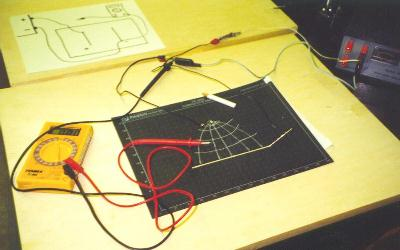AP Physics Lab

Mapping Electric Fields with Equipotential Lines

Introduction

Electric fields are produced around charged objects and are analogous to gravitational fields in several ways. However, since the electric field can attract or repel, an arbitrary positive test charge is used to determine the direction of the vector field, E.

Electric field lines extend outward from positively charged surfaces and end at negatively charged surfaces. The presence of conductors close to field lines can influence the direction of these lines, just like gravitational forces can be influenced by nearby masses such as planets.

In this lab, you will map an electric field under an atypical condition with either a conductor or insulator present. This will be done using equipotential lines on conductive paper. Recalling the potential energy of a charge in an electric field as U = q E d, and the more convenient measure of electric potential, U/q, or the volt, equipotential lines represent regions of equal potential energy at any distance from the electric field's source. Also, it becomes obvious that equipotential lines must run perpendicular to electric field lines, analogous to equal objects raised equal distances above a gravitational field.

Materials: conductive ink, voltmeter, conductive paper, probes

Procedures

1. Establish the pattern on the conductive paper as assigned using the conductive ink pen.2. Place your paper on the cork board, then hook up the electrodes and reference as indicated.

3. Apply a voltage of 5 - 20 V to the electrodes (conductive ink).

4. Begin mapping your equipotential field lines close to the negative terminal in intervals of 1 to 3 volts. The number of recordings should enable you to draw the general pattern of the equipotential field over the entire paper.

5. Since the equipotential field lines are perpendicular to the electric field lines, draw in the electric field lines using a different type marker. (NOTE: lines must not cross.)

Analysis

1. Your analysis consists of the completed electric field line vectors on the original conductive paper with a written presentation explaining the pattern your observed.

Back to the Brockport High School Science Department